# Flutter 绘制番外篇 - 圆中取形

#### 一、正 N 边形的绘制

##### 1. 正三角形绘制``````@override
void paint(Canvas canvas, Size size) {
canvas.translate(size.width / 2, size.height / 2);
int count = 3;
double radius = 140 / 2;
List<Offset> points = [];
for (int i = 0; i < count; i++) {
double perRad = 2 * pi / count * i;
}
_drawShape(canvas, points);
}````````````final Paint shapePaint = Paint()
..style = PaintingStyle.stroke;
void _drawShape(Canvas canvas, List<Offset> points) {
Path shapePath = Path();
shapePath.moveTo(points.dx, points.dy);
for (int i = 1; i < points.length; i++) {
shapePath.lineTo(points[i].dx, points[i].dy);
}
shapePath.close();
canvas.drawPath(shapePath, shapePaint);
}``````

##### 2. 正 N 边形

`正三角形` 同理，改变上面的 `count` 值，就可以将圆等分成 `count` 份，再对圆上对应点进行收集即可。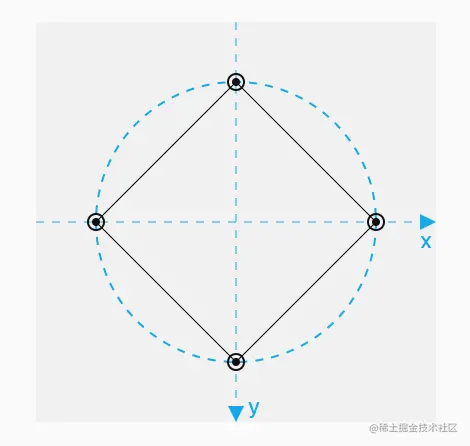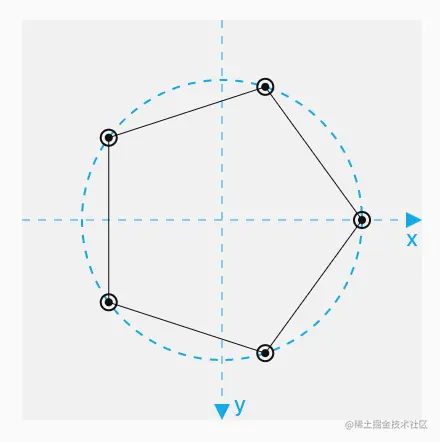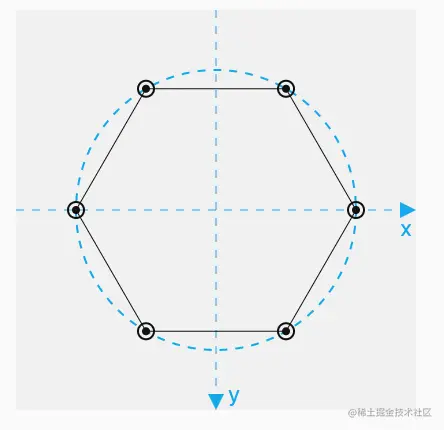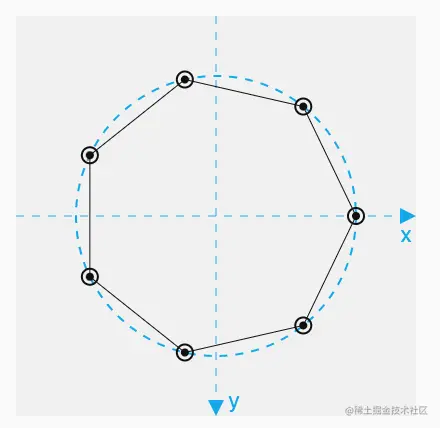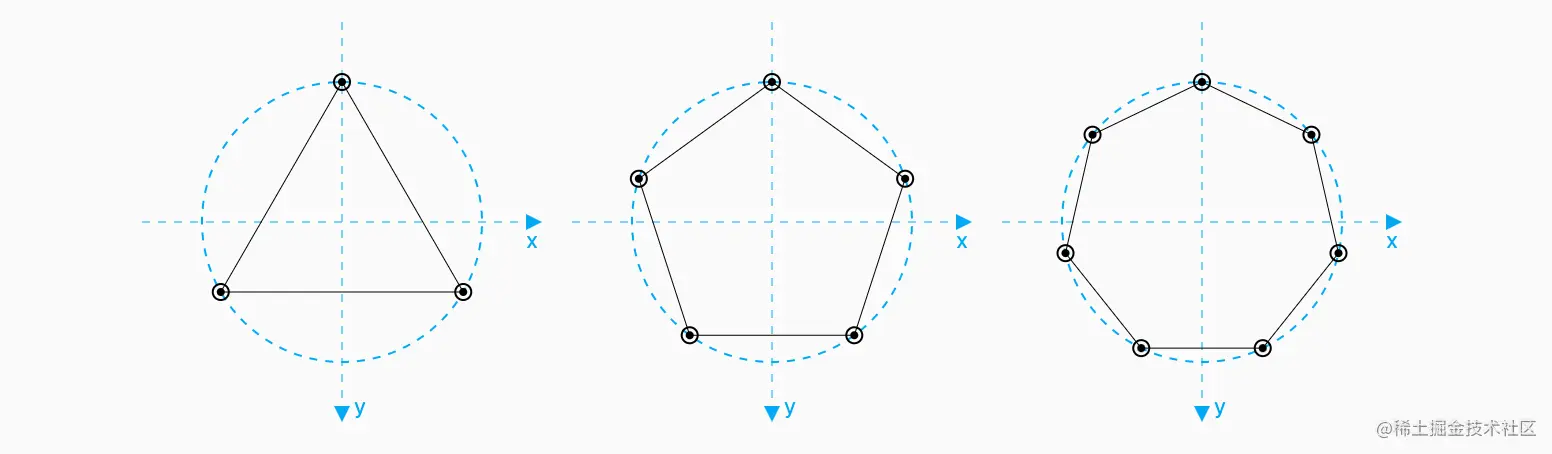``````double rotate = - pi / 2;
for (int i = 0; i < count; i++) {
double perRad = 2 * pi / count * i;
));
}``````

#### 二、 N 角星的绘制

##### 1、五角星的绘制``````for(int i =0; i< count; i++){
outPoints.insert(2*i+1, innerPoints[i]);
}````````````double innerRadius = 70 / 2;
List<Offset> innerPoints = [];
double offset = 15 * pi / 180;
for (int i = 0; i < count; i++) {
double perRad = 2 * pi / count * i;
));
}````````````List<Offset> innerPoints = [];
double offset = pi / count;
for (int i = 0; i < count; i++) {
double perRad = 2 * pi / count * i;
));
}``````

70/4070/2870/15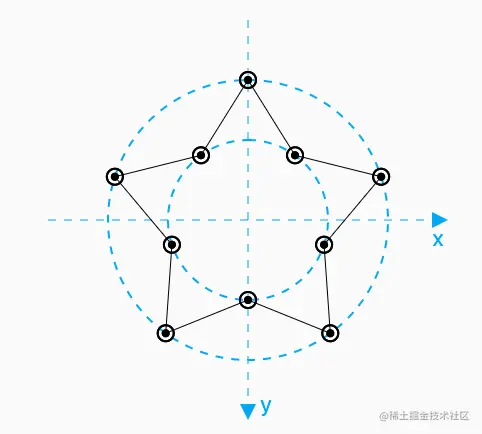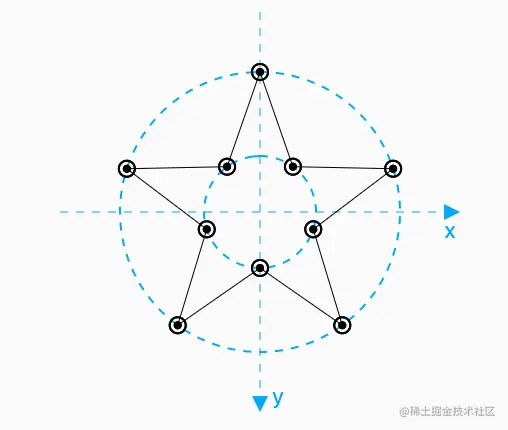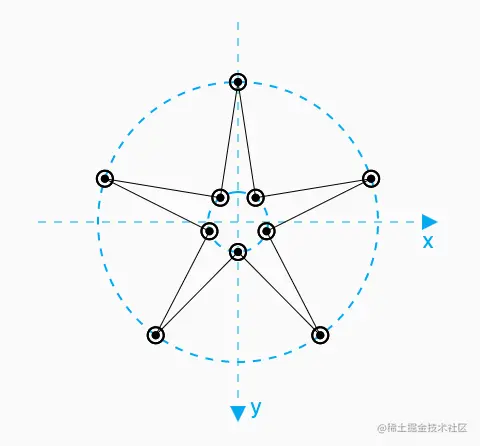##### 2. N 角星的绘制

``````α = 180°- 180°*(n-2)/n/2-90°
= 180°/n

``````int count = 6;
double outRadius = 140 / 2;
double innerRadius = 70 / 2;
double offset =  pi / count;
List<Offset> outPoints = [];
double rotate = -pi / 2;
for (int i = 0; i < count; i++) {
double perRad = 2 * pi / count * i;
));
));
}``````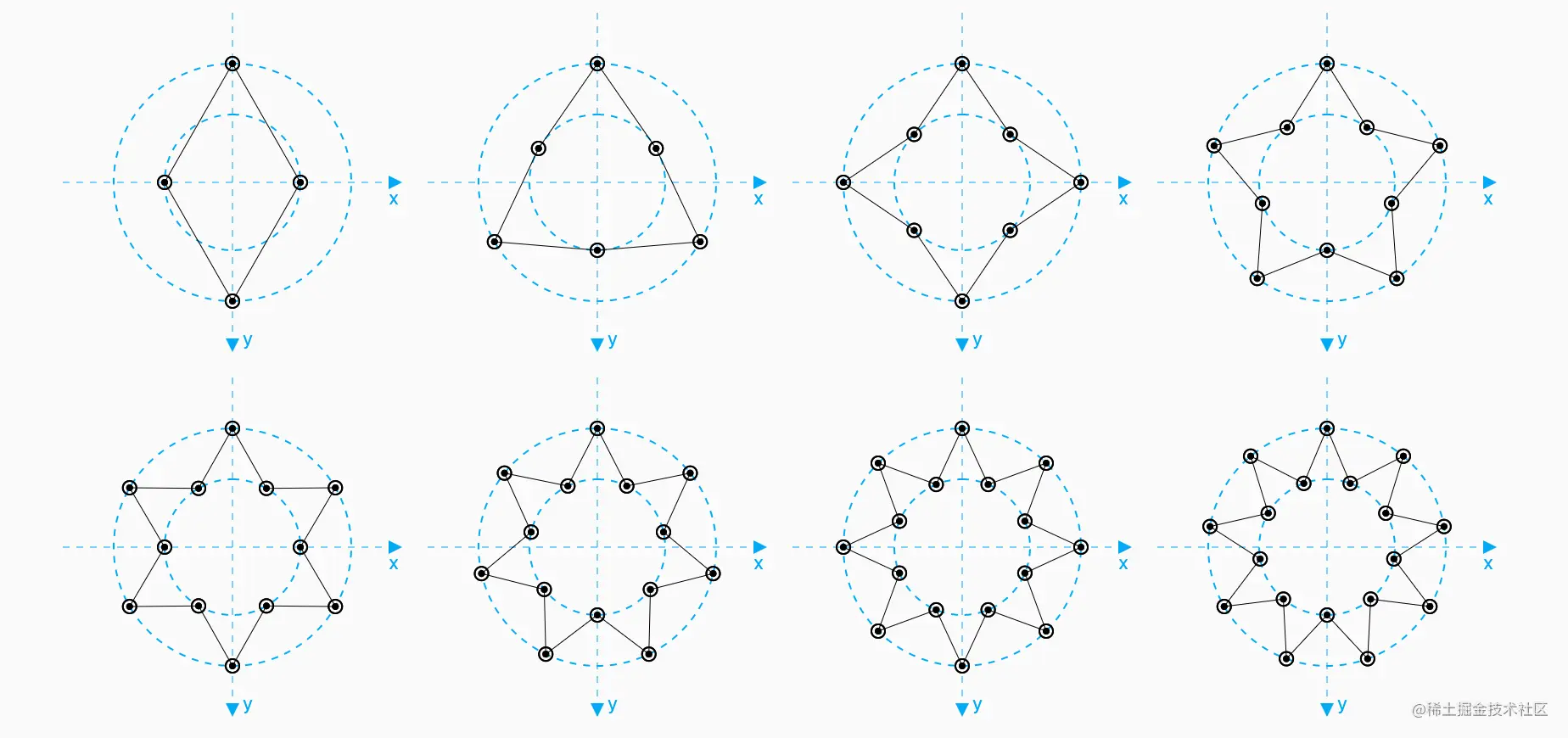#### 三、形状路径的使用

##### 1、路径工具的使用

``````// ShapePath型 成员变量
late ShapePath shapePath = ShapePath.star(
n: n,
);
// 获取 shapePath 中的路径
canvas.drawPath(shapePath.path, shapePaint);``````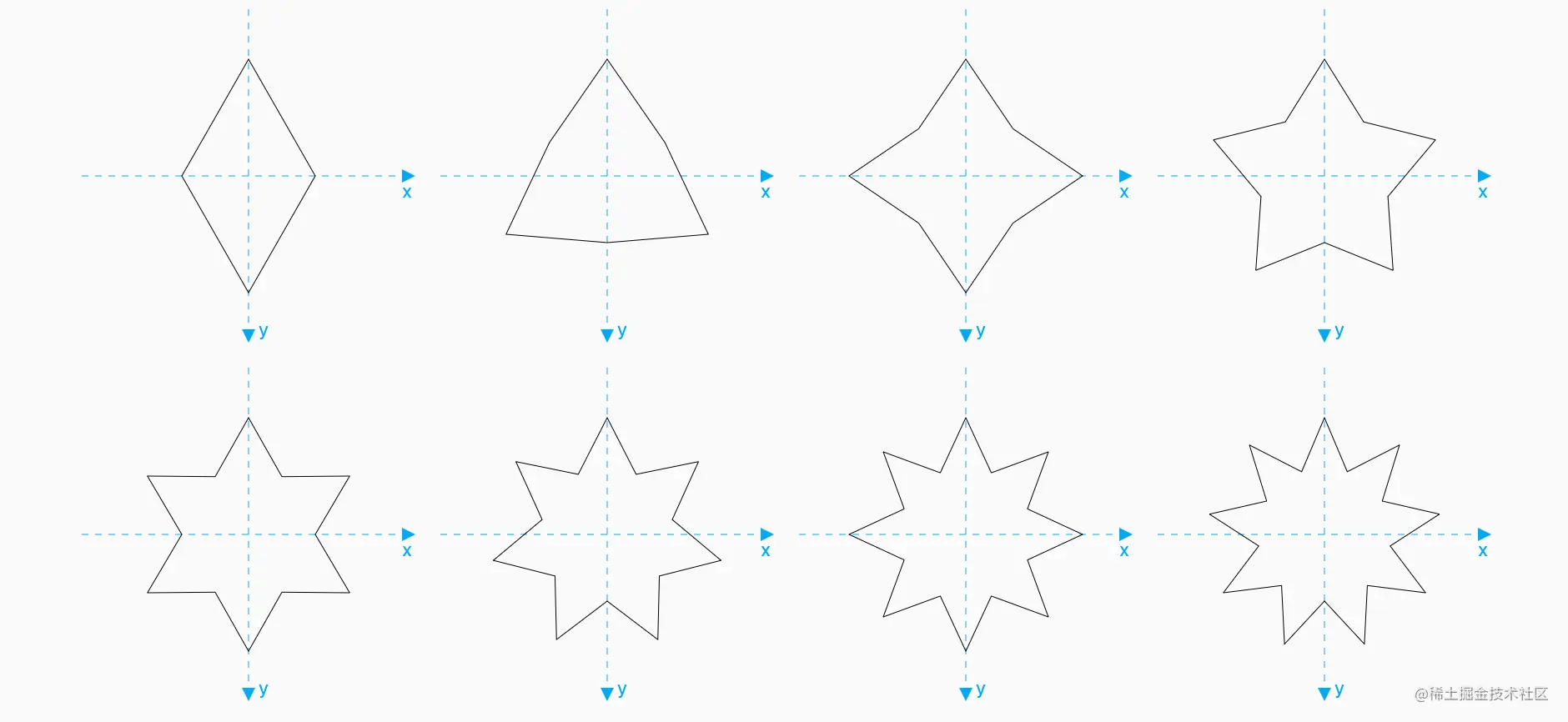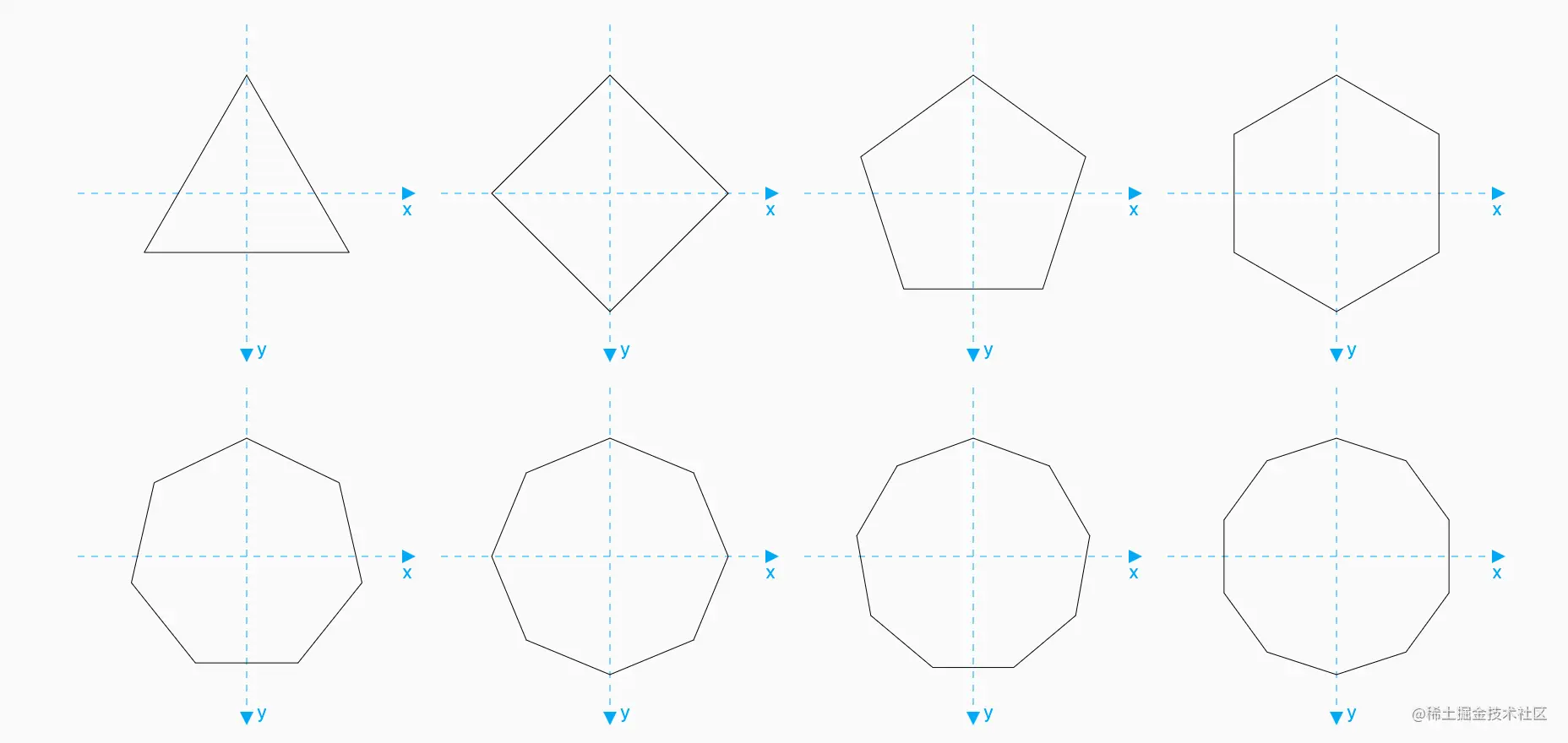##### 2、路径工具的封装

`ShapePath` 中有四个成员，其中 `n``outRadius``innerRadius` 是路径信息的配置，`_path` 是路径。在获取路径时做了个判断：如果路径为空，则先通过之前的逻辑构建路径，否则，直接返回已有路径。这样可以避免同一 `ShapePath` 对象构建多次相同的路径。

``````import 'dart:math';
import 'dart:ui';
class ShapePath {
ShapePath.star({
this.n = 5,
});
ShapePath.polygon({
this.n = 5,
final int n;
Path? _path;
Path get path {
if (_path == null) {
_buildPath();
}
return _path!;
}
void _buildPath() {
int count = n;
double offset = pi / count;
List<Offset> points = [];
double rotate = -pi / 2;
for (int i = 0; i < count; i++) {
double perRad = 2 * pi / count * i;
));
));
}
}
_path = Path();
_path!.moveTo(points.dx, points.dy);
for (int i = 1; i < points.length; i++) {
_path!.lineTo(points[i].dx, points[i].dy);
}
_path!.close();
}
}``````

##### 3、路径的作用

``````ClipPath(
clipper: ShapeBorderClipper(shape: MyShapeBorder()),
child: Image.asset(
'assets/images/wy_300x200.webp',
height: 200,
))````````````class MyShapeBorder extends ShapeBorder{
@override
EdgeInsetsGeometry get dimensions => const EdgeInsets.all(0);
@override
Path getInnerPath(Rect rect, {TextDirection? textDirection}) {
return Path();
}
@override
Path getOuterPath(Rect rect, {TextDirection? textDirection}) {
ShapePath shapePath = ShapePath.polygon(
n: 6,
);
return shapePath.path.shift(Offset(rect.longestSide/2,rect.shortestSide/2));
}
@override
void paint(Canvas canvas, Rect rect, {TextDirection? textDirection}) {
}
@override
ShapeBorder scale(double t) {
return this;
}
}``````